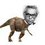# 1 equation , 2 variables.Solvable?

$\large a^3+b^3+3ab=1$

If $a,b \in R$ , Find value of $a+b$

Nice solutions are always welcome!Note by Nihar Mahajan
6 years, 3 months ago

This discussion board is a place to discuss our Daily Challenges and the math and science related to those challenges. Explanations are more than just a solution — they should explain the steps and thinking strategies that you used to obtain the solution. Comments should further the discussion of math and science.

When posting on Brilliant:

• Use the emojis to react to an explanation, whether you're congratulating a job well done , or just really confused .
• Ask specific questions about the challenge or the steps in somebody's explanation. Well-posed questions can add a lot to the discussion, but posting "I don't understand!" doesn't help anyone.
• Try to contribute something new to the discussion, whether it is an extension, generalization or other idea related to the challenge.

MarkdownAppears as
*italics* or _italics_ italics
**bold** or __bold__ bold
- bulleted- list
• bulleted
• list
1. numbered2. list
1. numbered
2. list
Note: you must add a full line of space before and after lists for them to show up correctly
paragraph 1paragraph 2

paragraph 1

paragraph 2

[example link](https://brilliant.org)example link
> This is a quote
This is a quote
    # I indented these lines
# 4 spaces, and now they show
# up as a code block.

print "hello world"
# I indented these lines
# 4 spaces, and now they show
# up as a code block.

print "hello world"
MathAppears as
Remember to wrap math in $$ ... $$ or $ ... $ to ensure proper formatting.
2 \times 3 $2 \times 3$
2^{34} $2^{34}$
a_{i-1} $a_{i-1}$
\frac{2}{3} $\frac{2}{3}$
\sqrt{2} $\sqrt{2}$
\sum_{i=1}^3 $\sum_{i=1}^3$
\sin \theta $\sin \theta$
\boxed{123} $\boxed{123}$

Sort by:

So , here is my trial:

$a^3+b^3+3ab-1=0 \\ (a+b)^3-3ab(a+b)+3ab-1=0 \\ (a+b)^3 - 3ab(a+b-1) -1=0 \\ (a+b)^3 - 1^3 = 3ab(a+b-1) \\ (a+b-1)(a^2+b^2+2ab+a+b+1)= 3ab(a+b-1)$

Let $a+b-1 \neq 0$

$\Rightarrow a^2+b^2+2ab+a+b+1= 3ab \\ a^2+b^2-ab+a+b+1=0 \\ a^2+b^2-(a-1)(b-1)=0 \\ a^2+b^2=(a-1)(b-1) \\ (a+b)^2 - 2ab = (a-1)(b-1) \\ (a+b+\sqrt{2ab})(a+b-\sqrt{2ab})=(a-1)(b-1) \\ \Rightarrow b+\sqrt{2ab} = -1 \quad a-\sqrt{2ab}=-1 \\ \Rightarrow a+b=-2 \quad a+b=1$

Is it right?

- 6 years, 3 months ago

yup, it's right

- 6 years, 3 months ago

Do like and reshare this for others (not for my sake). Thanks!

- 6 years, 3 months ago

Note @Mehul Arora @Vaibhav Prasad , $a,b$ are real numbers and not just integers. Cheers!

- 6 years, 3 months ago

Hey ,in the 5th step ,from where has -1 arrived in the second bracket I mean when you used the formula $a^{3} - b^{3}$ the correct equation should have been this $(a^{2} + b^{2} + 2ab +1 +a+b$)

- 6 years, 3 months ago

Sorry , it was a typing mistake. Thanks for correcting me.

- 6 years, 3 months ago

Please explain the second last step

- 6 years, 3 months ago

$(a+b+\sqrt{2ab})(a+b-\sqrt{2ab})=(a-1)(b-1) \\ \Rightarrow (a+(b+\sqrt{2ab}))(b+(a-\sqrt{2ab}))=(a-1)(b-1)$

If you compare the factors of $L.H.S$ and $R.H.S$ , correspondingly you will get:

$\Rightarrow b+\sqrt{2ab} = -1 \quad a-\sqrt{2ab}=-1$

- 6 years, 3 months ago

$\displaystyle a^3 + b^3 + c^3 -3abc = \dfrac{1}{2} (a+b+c)( (a-b)^2 + (b-c)^2 + (c-a)^2)$

Now substitute $c = -1$ and then L.H.S equals 0

$\displaystyle 0 = (a+b-1)( (a-b)^2 + (a+1)^2 + (b+1)^2)$

From here

$a+b = 1$ from the first and

$a+b = -2$ from second (easily solvable)

EDIT :- the second equation has only one solution when $a =-1$.

- 6 years, 3 months ago

Nice , that crucial substitution really helped!

- 6 years, 3 months ago

That identity is very useful it is just a result derived from another identity.

- 6 years, 2 months ago

The answer is $\space \boxed{a+b=1}$.

I plotted $f(a) = a^3+b^3+3ab-1$ for $b = -10, -7.5, 0, 7.5, 10$, thinking that there will be infinite solutions. But I discovered that the roots to $f(a)$ are respectively (a=11,8.5,1,-6.5,-9) making $\boxed{a+b = 1}$. See the graph here.- 6 years, 3 months ago

Sir , note that $a+b=-2$ is also a solution. See my and Krishna's solution. Thanks!

- 6 years, 3 months ago

There is one special case when $a+b=-2$.

- 6 years, 3 months ago

- 6 years, 3 months ago

What do you need help with? You solved the problem didn't you? :)

- 6 years, 3 months ago

Actually , I got it just after I posted this note. Since you have nice methods and approaches , I mentioned you.

- 6 years, 3 months ago

- 6 years, 3 months ago

I am not asking for the answer. I am asking for an elegant accurate solution with proof. -_-

- 6 years, 3 months ago

How about $a=-1$ and $b=-1$

- 6 years, 3 months ago

I am not asking for the answer. I am asking for an elegant accurate solution with proof. -_-

- 6 years, 3 months ago

See my bash , no need to compute $a,b$ :)

- 6 years, 3 months ago

Let a+b=x Now If, x=1, Then the Equation becomes a+b ^ 3 Thena+b=1 :D

P.S. I'm just too lazy. Idk What I am typing right now.

- 6 years, 3 months ago

That's right.

- 6 years, 3 months ago

I know.

- 6 years, 3 months ago

But that's incomplete. -_-

- 6 years, 3 months ago

Somewhat. But I laid the basis, didn't I ? :P

- 6 years, 3 months ago

Yeah you have a nice different idea. I am trying a different approach... xD

- 6 years, 3 months ago

Okay! So Cheers! xD

- 6 years, 3 months ago

See my bash , no need to compute $a,b$ :)

- 6 years, 3 months ago

a+b can be 1 also

- 6 years, 3 months ago

Yeah it can be $1$. So?

- 6 years, 3 months ago

LOL xD

- 6 years, 3 months ago